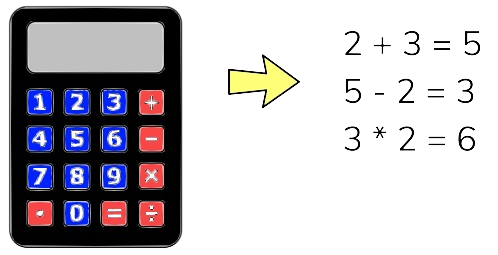# Project 005: Simple Integer Calculator

In this project, you will implement a simple integer calculator using C language. The calculator will have the following operators: addition, subtraction, multiplication, and division## Pin configurations

DevicePort.Pin Signal TypeModuleDirectionDrive Mode

You will use the following typedef enum to define the operators:

```typedef enum CAL_OPS{
CAL_SUB,
CAL_MUL,
CAL_DIV,
CAL_EQU,
CAL_CLR
} CAL_OPS;```

You will also use a struct to define the variables of the calculator, as follows:

```typedef struct CALULATOR{
int     currValue;
int     result;
CAL_OPS opCode;
} CALCULATOR;
CALCULATOR  cal;
```

You will declare an instance of the calculator as follows

```CALCULATOR  cal;
```

You will implement the following functions to handle the calculator response:

• void Cal_Clear()
This function will clear the calculator's current value (cal.currValue) and result (cal.result) to 0 and set the operator (cal.opCode) to addition (CAL_ADD). Then, it will display the result on the LCD screen.
• void Cal_DigitIn(int din)
This function will take an integer digit (din) ranging from 0 to 9 as input. It will add the digit to the current value (cal.currValue) and display it on the LCD screen.
• void Cal.OperatorIn(CAL_OPS op)
• This function will take an operator code (op) as input. If op is one of the arithmetic operators (CAL_ADD, CAL_SUB, CAL_MUL, or CAL_DIV), it performs the corresponding calculation using the current operator code (cal.opCode) and the current value (cal.currValue), and updates the result accordingly (result = result opCode currValue). Then, it resets the current value (cal.currValue) to 0 and sets the operator code (cal.opCode) to the new input operator code (op). Finally, it displays the result on the LCD screen.

For example, if the current value is 5, the current operator code is CAL_ADD, and the input operator code is CAL_SUB, the function will perform the following calculation: result = result + currValue = 0 + 5 = 5. Then, it will reset the current value to 0 and set the operator code to CAL_SUB. The LCD screen will display the result 5.
• If the input operator code (op) is the equal operator (CAL_EQU), the function will perform the calculation using the current operator code (cal.opCode), the current value (cal.currValue), and updates the result accordingly (result = result opCode currValue). After the calculation, it will reset the current value (cal.currValue) to 0 and set the operator code (cal.opCode) to CAL_ADD. Finally, it will display the result on the LCD screen.
• If op is the clear operator (CAL_CLR), the function calls the Cal_Clear() function to clear the calculator's values and display 0 on the LCD screen.

After setting up the peripherals in your embedded code, call the Cal_Clear() function to initialize the calculator. In the infinite loop, read the keypad every 20ms using the ReadKeypad() function.

• If the ReadKeypad() function returns a digit key ('0' to '9'), call the Cal_DigitIn() function with the corresponding digit as the input parameter.
• If the ReadKeypad() function returns the 'A' key, call the Cal_OperatorIn() function with the CAL_ADD operator code as the input parameter.
• If the ReadKeypad() function returns the 'B' key, call the Cal_OperatorIn() function with the CAL_SUB operator code as the input parameter.
• If the ReadKeypad() function returns the 'C' key, call the Cal_OperatorIn() function with the CAL_MUL operator code as the input parameter.
• If the ReadKeypad() function returns the 'D' key, call the Cal_OperatorIn() function with the CAL_DIV operator code as the input parameter.
• If the ReadKeypad() function returns the '*' key, call the Cal_OperatorIn() function with the CAL_CLR operator code as the input parameter.
• If the ReadKeypad() function returns the '#' key, call the Cal_OperatorIn() function with the CAL_EQU operator code as the input parameter.

By implementing these functions, you will be able to create a simple integer calculator that can perform basic arithmetic operations. Good luck!